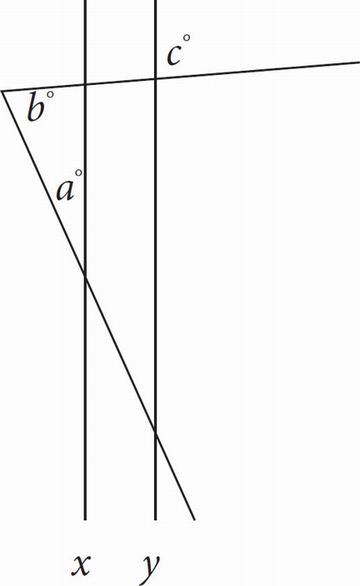# SAT Math Multiple Choice Question 58: Answer and Explanation

### Test Information

Question: 58

4.In the figure above, x || y. What is the value of a ?

• A. b + c
• B. 2b - c
• C. 180 - b + c
• D. 180 - b - c